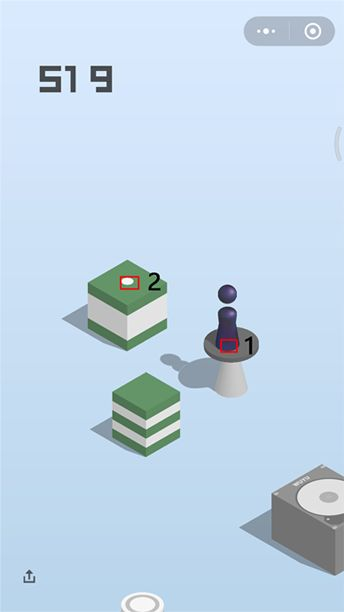# 用 Python 从零开始玩微信跳一跳

2、通过点击图上两个坐标点，算出两点之间的距离（勾股定理），最后得出按压屏幕3的时间。

4、重复 1 – 3

1. `import os`
2. `import math`
3. `# 这两个库用于图片的操作`
4. `import matplotlib.pyplot as plt`
5. `from PIL import Image`

1. `class WechatJump:`
2. `    def __init__(self):`
3. `        # 按压系数，不同分辨率的手机需要做调整`
4. `        self._coefficient = 1.35`
5. `        # 记录按压次数`
6. `        self._click_count = 0`
7. `        # 记录点击点坐标的数组`
8. `        self._coords = []`

1. `def generate_screenshot(self):`
2. `    # 截图，并将图片保存为 /sdcard/screenshot.png`
3. `    os.system('adb shell screencap -p /sdcard/screenshot.png')`
4. `    # pull 命令是将图片从手机发送到电脑本地`
5. `    os.system('adb pull /sdcard/screenshot.png .')`

1. `# event 是点击事件`
2. `def on_click(self, event):`
3. `    # event.xdata, event.ydata 分别是点击的横纵坐标，将坐标依次保存到 _coords 数组中`
4. `    self._coords.append((event.xdata, event.ydata))`
5. `    # 这里是每两次点击（起始点和目标点）就会执行按压按压屏幕操作，所以当`
6. `    # self._click_count == 2 时才执行`
7. `    self._click_count += 1`
8. `    if self._click_count == 2:`
9. `        self._click_count = 0`
10. `        # 弹出第二次点击时的坐标`
11. `        _next = self._coords.pop()`
12. `        # 弹出第一次点击时的坐标`
13. `        _prev = self._coords.pop()`
14. `        # 根据勾股定理计算出两点之间的距离`
15. `        self.jump_to_next(`
16. `            math.sqrt((_next - _prev) ** 2 + (_next - _prev) ** 2))`1. `def jump_to_next(self, distance):`
2. `    press_time = int(distance * self._coefficient)`
3. `    # cmd 最后一个参数 press_time 为按压时间，按压时间为 距离x按压系数，至于按压系数是`
4. `    # 多少则要根据每个人的手机分辨率而定，可自行测试调整`
5. `    # 100 100 200 200 这四个数字其实无所谓，只是模拟 swipe 操作时的坐标点而已`
6. `    cmd = 'adb shell input swipe 100 100 200 200 {}'.format(press_time)`
7. `    print(cmd)`
8. `    # 执行命令`
9. `    os.system(cmd)`

1. `def run(self):`
2. `    # 循环执行操作`
3. `    while True:`
4. `        # 这里执行两次截图操作，不然会提示远程图片未找到的问题（可以注释其中一行试试看）`
5. `        self.generate_screenshot()`
6. `        self.generate_screenshot()`
7. `        figure = plt.figure()`
8. `        # 绑定 on_click 操作`
9. `        figure.canvas.mpl_connect('button_press_event', self.on_click)`
10. `        # 打开并显示图片`
11. `        img = Image.open('screenshot.png')`
12. `        plt.imshow(img)`
13. `        plt.show()`

1. `wechat_jump = WechatJump()`
2. `wechat_jump.run()`